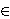### Zvonimir Janko

Mathematical Institute, University of Heidelberg, 69120 Heidelberg, Germany
e-mail: janko@mathi.uni-heidelberg.de

Abstract.   We determine up to isomorphism finite non-Dedekindian p-groups G (i.e., p-groups which possess non-normal subgroups) such that the normal closure of each non-normal cyclic subgroup in G is nonabelian. It turns out that we must have p=2 and G has an abelian maximal subgroup A of exponent 2e, e≥ 3, and an element vG-A such that for all hA we have either hv=h-1 or hv=h -1+2e-1.

2010 Mathematics Subject Classification.   20D15.

Key words and phrases.   Finite p-groups, normal closure, quasidihedral 2-groups, quasi-generalized quaternion groups, exponent of a p-group.

Full text (PDF) (access from subscribing institutions only)

DOI: 10.3336/gm.49.2.07

References:

1. Y. Berkovich, Groups of prime power order, Vol. 1, Walter de Gruyter, Berlin-New York, 2008.
MathSciNet

2. Y. Berkovich and Z. Janko, Groups of prime power order, Vol. 3, Walter de Gruyter, Berlin-New York, 2011.
MathSciNet

3. Y. Berkovich and Z. Janko, Groups of prime power order, Vol. 5, Walter de Gruyter, Berlin-New York, 2014.